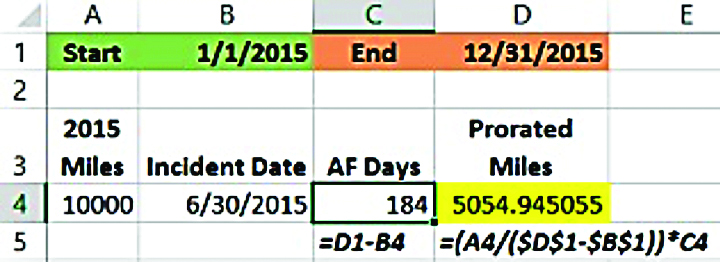Days in Excel formulasby on
in

Q. I need to prorate mileage over the number of days an employee was accident free. What would that formula look like?

A. Well, it’s actually a couple of formulas. First you need to know the number of days they were accident free. To accommodate a possible leap year, you may want to use start and end dates as I have in the green- and orange-shaded cells. The end-of-the-year date minus the accident date would yield the new number of days accident free (D1-B4). To be precise you may want to add 1.

Now that we know that, we can divide the total miles driven for the year by the number of days in the year. While you could use 365, or 360, if you want to be precise, subtract the start date for the year (green) from the end date for the year (orange).Now, multiply that by the number of accident-free days. Use an absolute cell reference for the start and end dates to make sure they don’t “travel” as you copy them down (\$B\$1, \$D\$1).